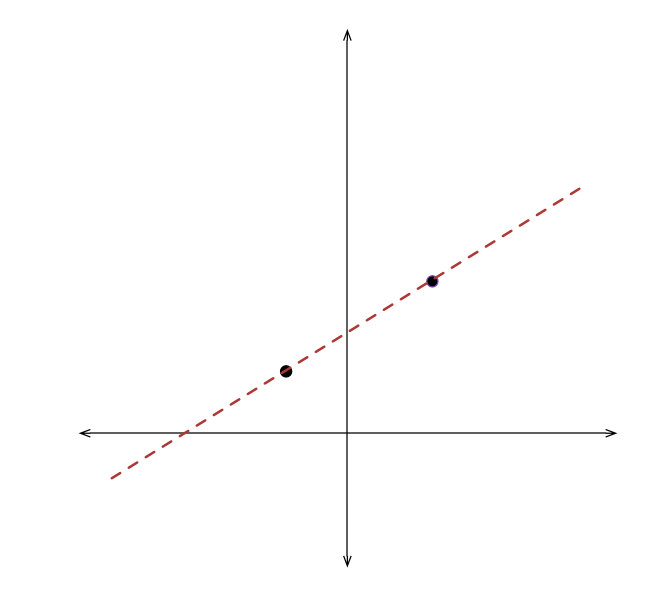# Coordinates

Geometry Level 1In a coordinate plane, if the points C(2,5) , D(-1,2) and E(x,y) lie on line l, which of the following could be the coordinates of point E?

×